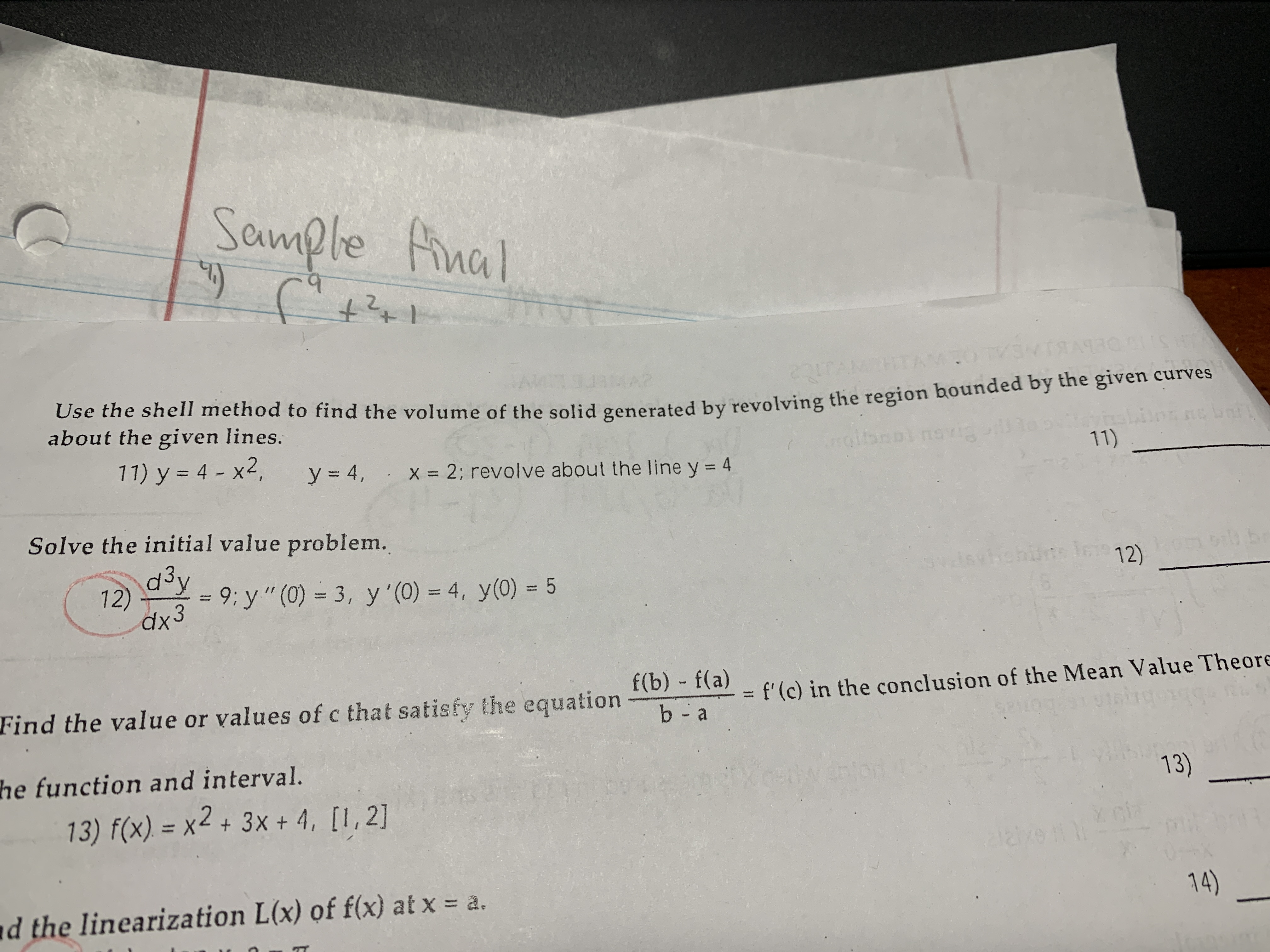# Sample nal+2+A2Use the shell method to find the volume of the solid generated by revolving the region bounded by the given curvesabout the given lines.11) y = 4 - x2,SLCnsviay= 4,x 2; revolve about the line y = 411)Solve the initial value problem.d3yIcregmbib bJ5thobudgic 12)12)dx39: y" (0) 3, y'(0) 4, y(0) 5f(b) f(a)Find the value or values of c that satisfy the equationf'(c) in the conclusion of the Mean Value Theoreb - ahe function and interval.or13)13) f(x) = x2+ 3x + 4, [I,2]clad the linearization L(x) of f(x) at xa.14)

Question
2 views

Can you help me solve and understand number 11. (Shell method) thanks!help_outlineImage TranscriptioncloseSample nal +2+ A2 Use the shell method to find the volume of the solid generated by revolving the region bounded by the given curves about the given lines. 11) y = 4 - x2, SLC nsvia y= 4, x 2; revolve about the line y = 4 11) Solve the initial value problem. d3y Icre gmbib b J5thobudgic 12) 12) dx3 9: y" (0) 3, y'(0) 4, y(0) 5 f(b) f(a) Find the value or values of c that satisfy the equation f'(c) in the conclusion of the Mean Value Theore b - a he function and interval. or 13) 13) f(x) = x2+ 3x + 4, [I,2] cla d the linearization L(x) of f(x) at x a. 14) fullscreen
check_circle

Step 1

we graph the region first.

Step 2

h=height = 2-x=2-sqrt(4-y)

Step 3

Put them in the sh...

### Want to see the full answer?

See Solution

#### Want to see this answer and more?

Solutions are written by subject experts who are available 24/7. Questions are typically answered within 1 hour.*

See Solution
*Response times may vary by subject and question.
Tagged in